### Final Project Week 9 - Recoil Implementation

Earlier this week I made a blog post about the research I have done about recoil in other games. In this post, I will be taking what I have learned from that research and implementing it into my system.

### Ensuring shots go where expected

The first thing I wanted to do before I implemented any form or recoil into the system, was to ensure that the shots are going exactly where expected. I have been thinking about how I would go about this for a while. Previously, the shots are fired from the centre of the screen, if the deviation is turned on, they will be calculated from the centre of the screen. My system will be used by people that will not want to tweak different values for a long time to make sure that the reticle of their scope is perfectly aligned with the centre of the screen. Because of this, I decided that scopes will have to include an empty game object that will function as the reticle centre. Wherever this is, the shot will be centred on it. This ensures that no matter what, the shot will always go exactly where you would expect. Below is an example of the change, I have purposely given the weapon a large offset to show the new feature.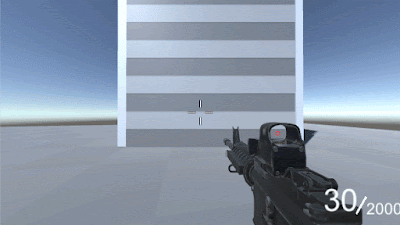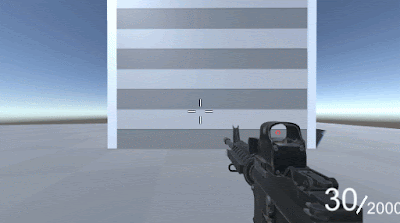The old method always calculates shots from the centre of the screen The new method calculates shots relative to the reticle

### Calculating the next recoil position

The first step towards making the system is deciding where the next shot will go. to do this I want to use a similar system to Rainbow Six Siege. In their video, they mention that they use a diamond to determine the next point. I want the recoil in my system to be very flexible so that developers can make it match exactly what they want, but I don't want it to have so many variables that it becomes confusing. To achieve this I have created 5 variables that the developer can change.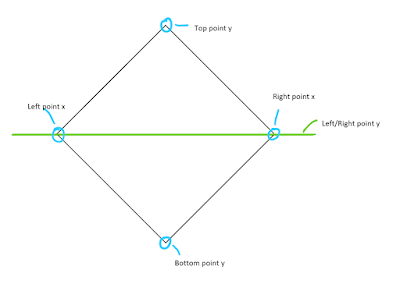Illustration of the different variables of the diamond

The variables I have created are the Top Point y, Bottom Point y, Left Point x, Right Point x, and Left/Right Point y. These values can be utilised to create a wide variety of recoil patterns that can be used to fit a lot of games.

To pick a random point within my diamond requires first splitting it into 2 triangles. Because of this, I start creating the diamond by creating 2 triangles, one for the left of the diamond, one for the right. the points of these are determined by the values given in the inspector.

The next step to picking a point requires picking one of the triangles at random. when picking, the system must take into account the variables that the developer has input. this means that the area of each triangle is calculated and added together. It then generates a random number between 0 and the total area, if the random area is more than the area of the left then the right triangle is picked.

Next, a random point within the selected triangle is selected. I found an equation at wolfram.com that will allow me to pick a random value within the area of a triangle.

a1 and a2 are random numbers between 0 and 1
v1 and v1 and v2 are2 points on the triangle relative to the 3rd point.
x = a1v1 + a2v2

A downside to this equation is that it is used to find a random point within a quadrilateral, a simple solution that I found to fix this, is to check the sum of a1 and a2. If the sum is over 1, it will not fit within the triangle. My original solution to this was to continuously pick a number until the sum was less than or equal to 1. The issue with this is that the random numbers being generated could continuously add up to over 1. This means that it is quite inefficient as it could be needlessly generating random numbers and writing memory.Using a while loop can cause unnecessary calculations

The solution that I went with in the end was much simpler and more efficient. Now, the numbers are randomly generated only once, then if the sum totals over 1, it will subtract each value from 1 and set that as the new value.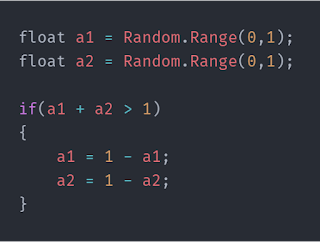Using this method will ensure that the numbers are never changed more than once

Now that a random point was able to be picked, it was time to move onto translating these random points into actual weapon recoil. The way I plan for this to work is; as the weapon shoots, the camera will move to look at the random point, the weapon is then moved as a visual effect, this the developer would be able to configure. When the user stops shooting, the camera will return to the start point, with some adjustments made while the user is adjusting for recoil.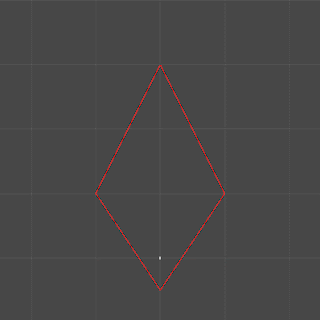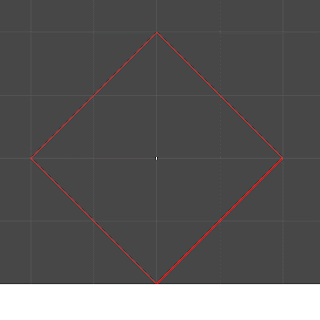### Applying the maths to the gun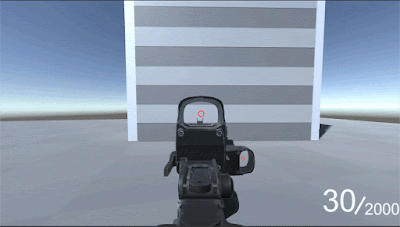Instantly snapping to the next recoil point
To start, I just wanted to make the camera snap to the chosen recoil, I can then smooth out that movement once it works. To move the camera, I made it calculate the next point by using a similar method as last weeks bullet deviation method. I raycast from the screen point relative to the centre of the screen plus the new recoil point. I then use transform.LookAt(), which will snap the camera to looking at the new recoil point.Moving between the points smoothly
To smooth this out, I lerp between the current point and the look at point, using a speed that is on the weapon so that the developer can configure it. I made the value relating to the amount of time you would like it to take to get to the next recoil point to make the system easier to understand.

Next week I will be refining the recoil system, adding a return after shooting and further smoothing out the movement.

You can find the project on GitHub here: https://github.com/ElliotChester/Modular-Weapon-System-Final-Project

## References

Battlefield V. 2018. PC [Game]. EA Dice

Apex Legends. 2019. PC [Game]. Respawn Entertainment

Rainbow Six Siege. 2015. PC [Game]. Ubisoft

Bansal, A. (2018). Triangles - Everything about formulas and areas of these polygons!. [online] Toppr Bytes. Available at: https://www.toppr.com/bytes/triangles/ [Accessed 15 Feb. 2019].

Evernden, S. (2008). How can I determine whether a 2D Point is within a Polygon?. [online] Stack Overflow. Available at: https://stackoverflow.com/questions/217578/how-can-i-determine-whether-a-2d-point-is-within-a-polygon [Accessed 15Feb. 2019].

Jaspelkis, A. (2013). How to get a random point on the interior of an irregular polygon?. [online] Stack Overflow. Available at: https://stackoverflow.com/questions/19481514/how-to-get-a-random-point-on-the-interior-of-an-irregular-polygon [Accessed 20 Feb. 2019].

Weisstein, E. (2019). Triangle Point Picking. [online] Mathworld.wolfram.com. Available at: http://mathworld.wolfram.com/TrianglePointPicking.html [Accessed 15 Feb. 2019].